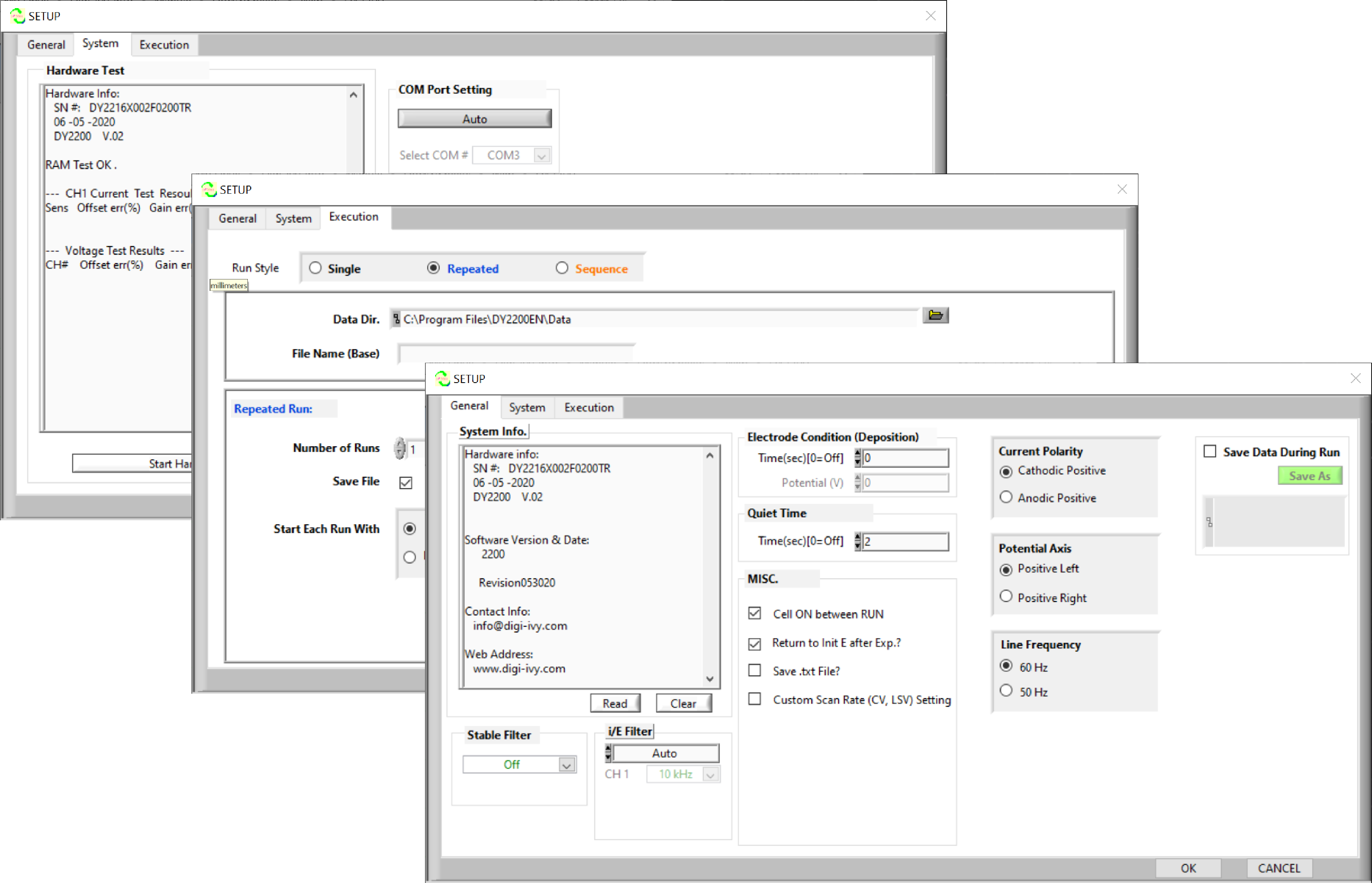## DY2200 Series Mini Potentiostat/Galvanostat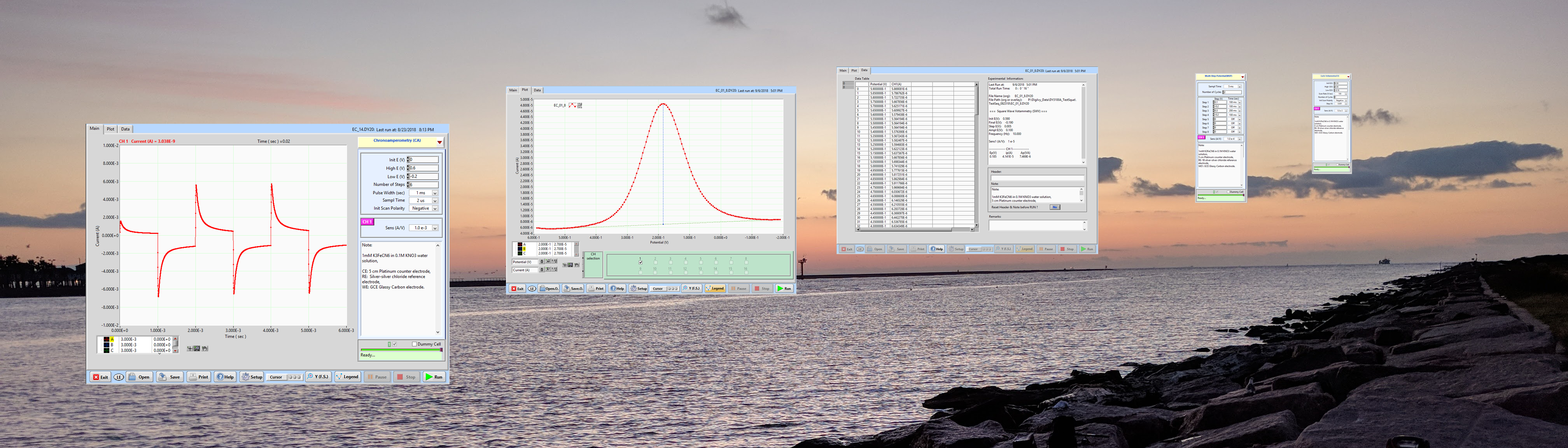## Hardware

 Electrode Configurations 3 (CE, RE, WE) with cell On/Off control Current Range (Potentiostat) ±100.0 nA to ±10.0 mA (full scale) in 6 steps Current Range (Galvanostat) ±1.0 nA to ±10.0 mA Max. CV Scan Rate 100V/sec Min. Sampling Time 2 µsec Current Resolution 0.002% of full scale, with highest resolution at 3 pA Input Impedance of Electrometer > 1012 Ω Potential Range ±4.0 V (16-bit DAC) Potential Bandwidth > 100 kHz Compliance Voltage ≥ ±10 V I/E Low Pass Filter 6 ranges (Auto or Manual), depend on sensitivity setting Input Bias Current < 30 pA @ 25 °C ADC Sampling 16-bit (60000 data max.) Dimensions & Weight 7 x 14 x 3 cm, 260g Power Requirements USB powered

## Software

• Easy-to-use user interface for experimental setup, graphic display, data analysis and file management
• Data Processing (Filter, Smoothing, Remove DC Offset, Math, FFT, Tafel Plot, etc.)
• Automatic peak potential, current reporting and charge calculation
• Run style: _Single, Auto Repeat or Auto Sequence_
• Plots overlay and text data exportation
• Easy cursors define and measurement
• Real time display with Pause and Stop Exp. functions
• USB connection to a user-provided PC running Windows

• ## DY2200 Series Models:

Function \ Model Number DY2211 DY2213 DY2216
Amperometric i-t (iT)
Cyclic Voltammetry (CV)
Linear Sweep Voltammetry (LSV)
Open Circuit Potential vs. Tim (OCP)
Differential Pulse Voltammetry (DPV)
Normal Pulse Voltammetry (NPV)
Multi-Step Potential (MSP)
Square Wave Voltammetry (SWV)
Chronoamperometry (CA)
Potentiometric V-t (V-t)
Chronopotentiometry (CP)
Chronopotentiometry with Current Ramp (CPCR)
Multi-Step Current (MSC)

## Software Techniques (Potentiostat/Galvanostat)

 Amperometric i-t curve (iT) Sampling Time (sec) = [2e-6 to 100] Cyclic Voltammetry (CV) Scan Rate (V/sec) = [1e-5 to 100] Linear Sweep Voltammetry (LSV) Scan Rate (V/sec) = [1e-5 to 100] Open circuit potential vs. time (OCP) Sampling Time (sec) = [2e-6 to 100] Differential Pulse Voltammetry (DPV) Step E (V) = [0.001 to 0.1], Amplitude (V) = [0.001 to 0.5], Pulse Period (sec) = [0.001 to 100] Normal Pulse Voltammetry (NPV) Step E (V) = [0.001 to 0.5], Pulse Period (sec) = [0.001 to 100] Multi-Step Potential (MSP) Sampling Time (sec) = [2e-6 to 20], Step E (V) = [-4.0, +4.0], Step Width (sec) = [0.001 to 200] Square Wave Voltammetry (SWV) Step E (V) = [0.001 to 0.1], Frequency (Hz) = [0.01 to 1000] Chronoamperometry (CA) Sample Time (sec) = [2e-6, 20] , Number of Steps = [2, 1000], Pulse Width (sec) = [0.001, 1000] Potentiometric V-t (V-t) Current (A) = [1e-9, 0.01], Sampling Time (sec) = [2e-6 to 100] Chronopotentiometry (CP) Step Current (A) = [1e-9, 0.01], Step Time (sec) = [1e-3, 10000], Sample Time (sec) = [2e-6,100] Chronopotentiometry with Current Ramp (CPCR) Current (A) = [1e-9, 0.01], Scan Rate (A/sec) = [2e-9, 1.0] Multi-Step Current (MSC) Sampling Time (sec) = [2e-6 to 20], Step i (A) = [-0.01, +0.01], Step Width (sec) = [0.001 to 200]

## Sample Plots

•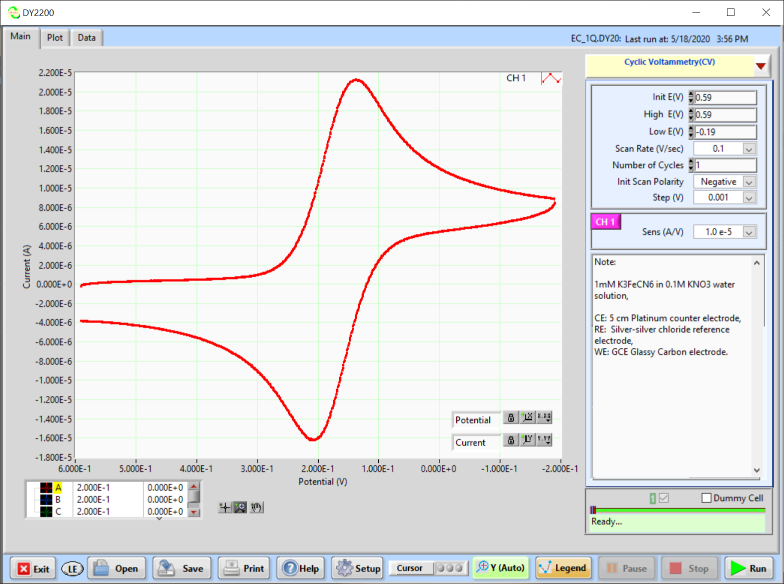•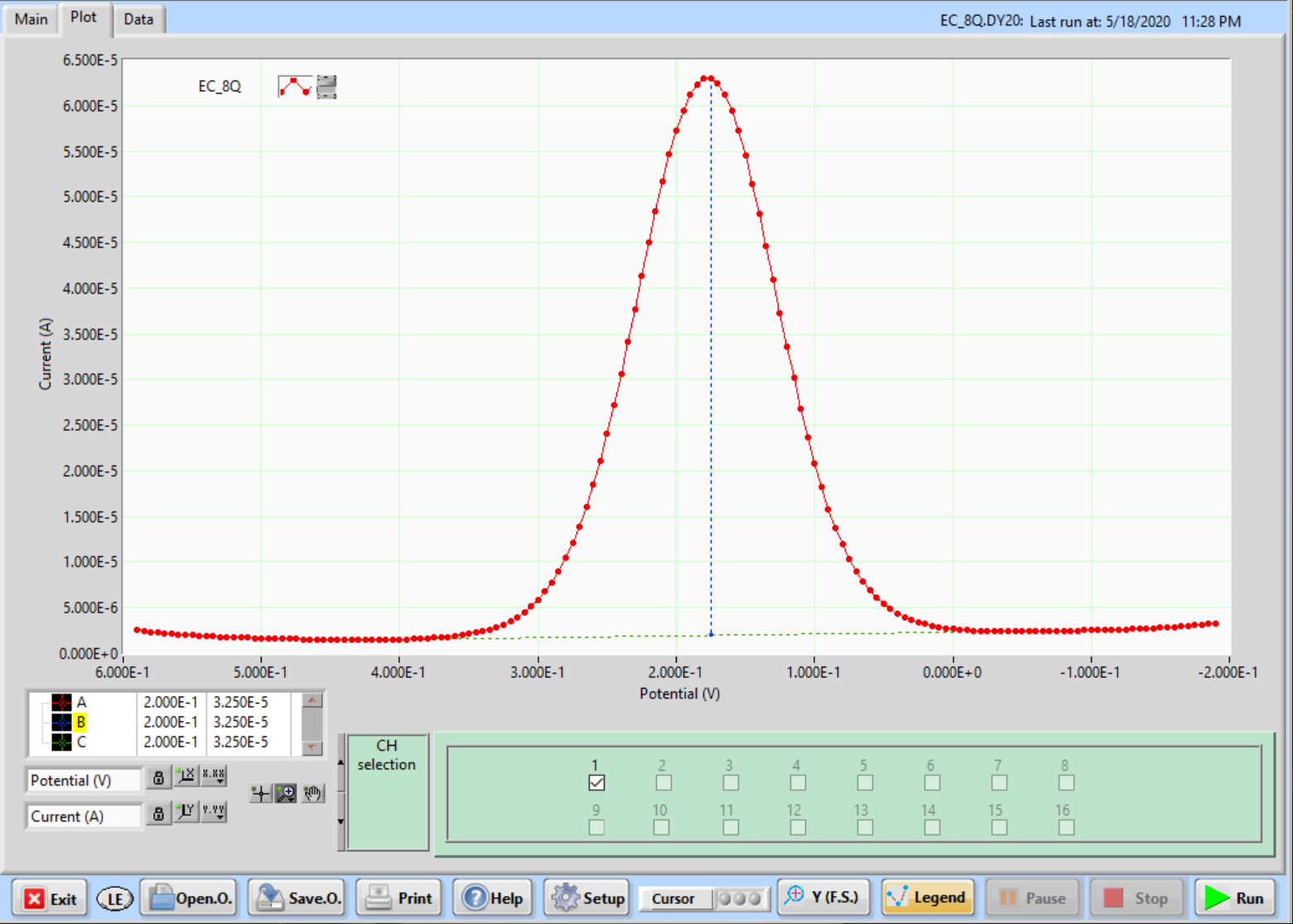•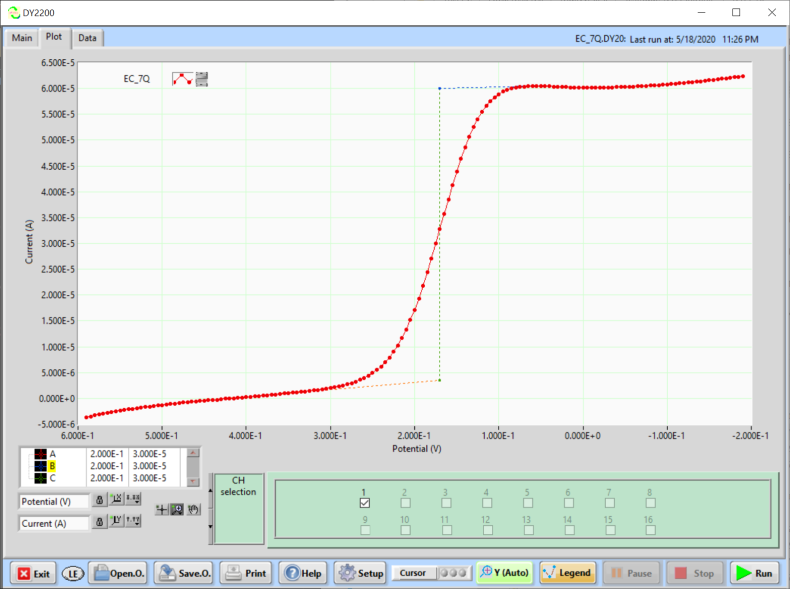•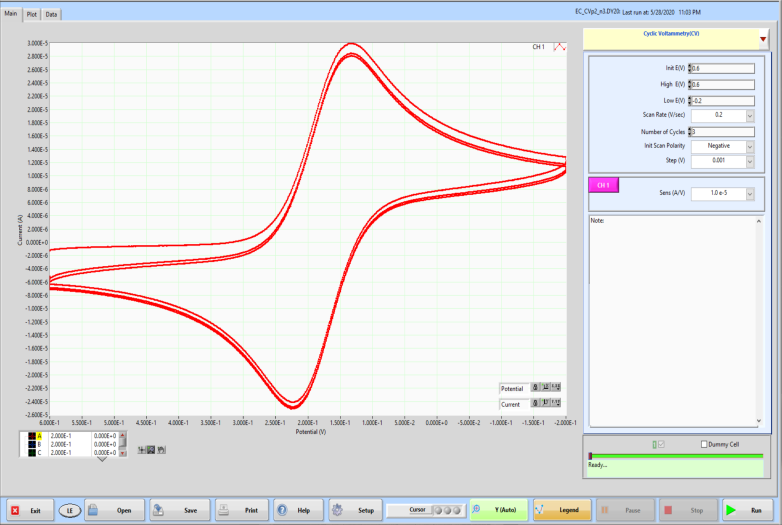•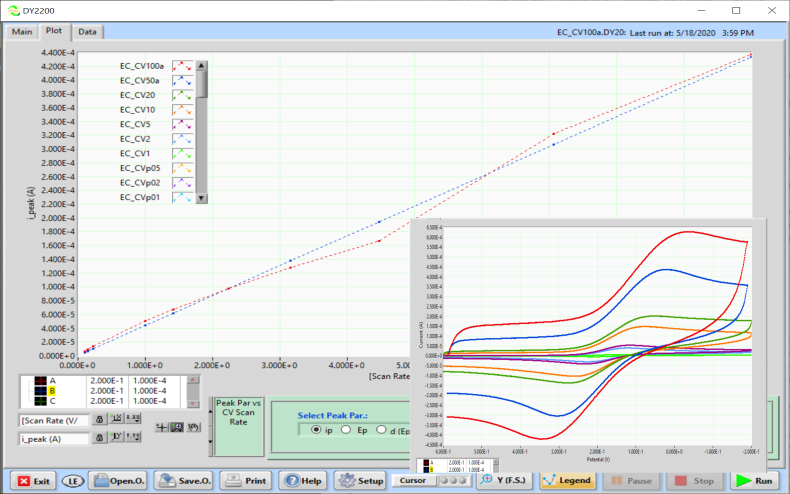•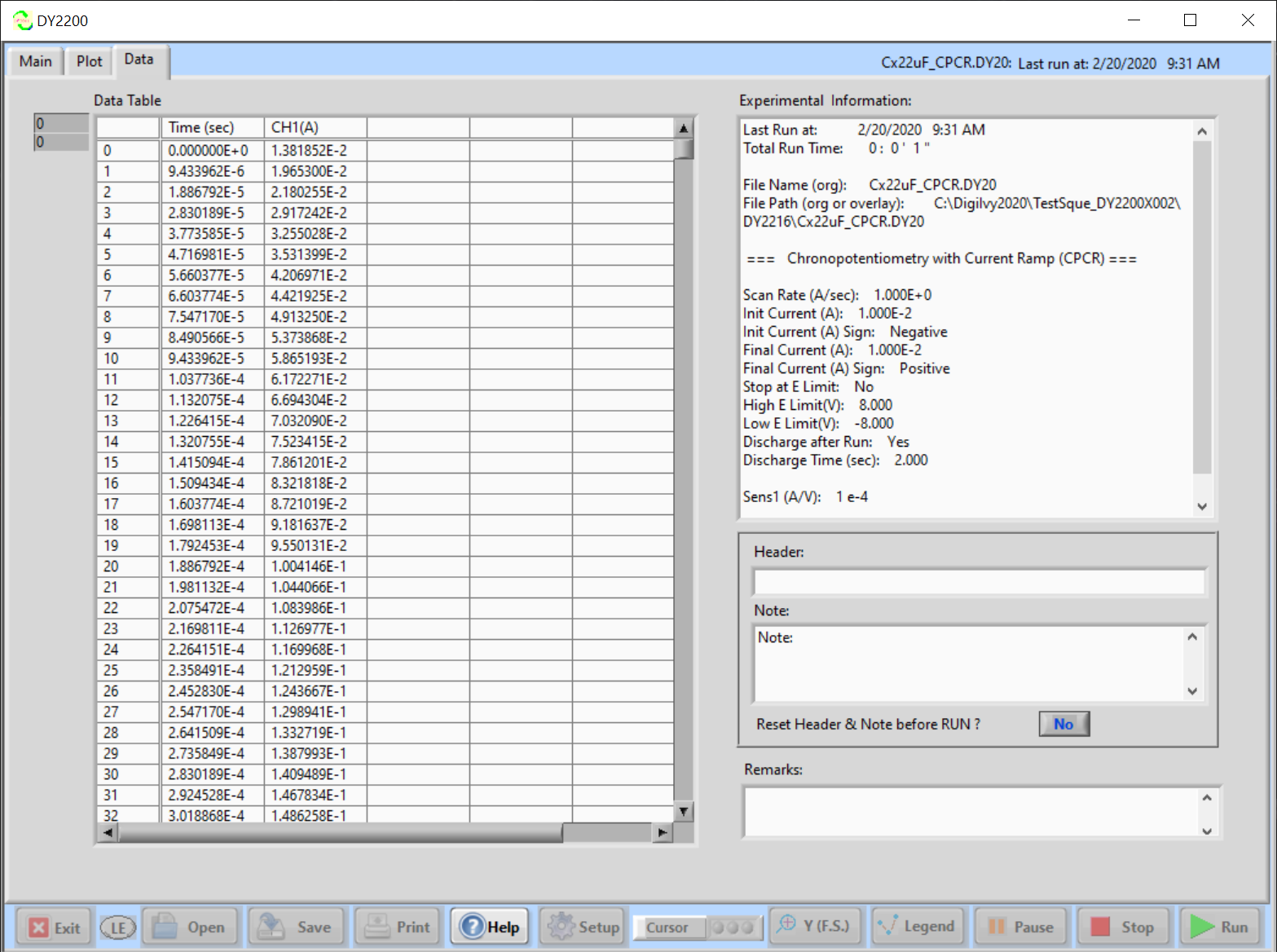•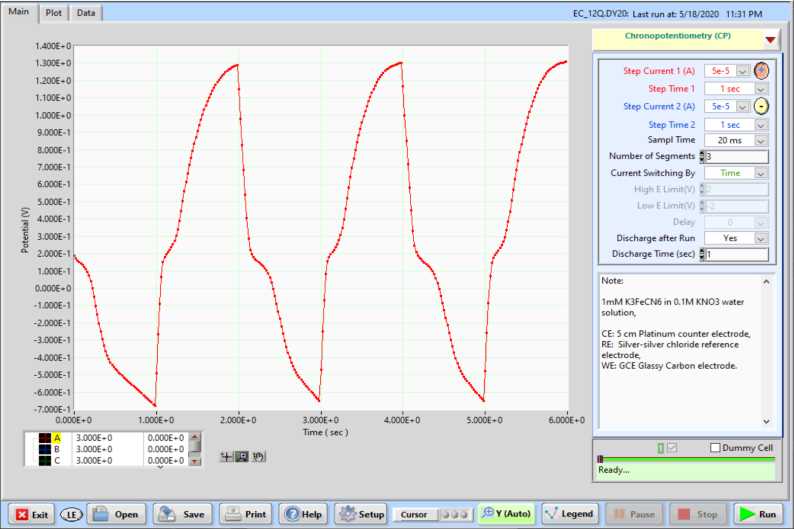•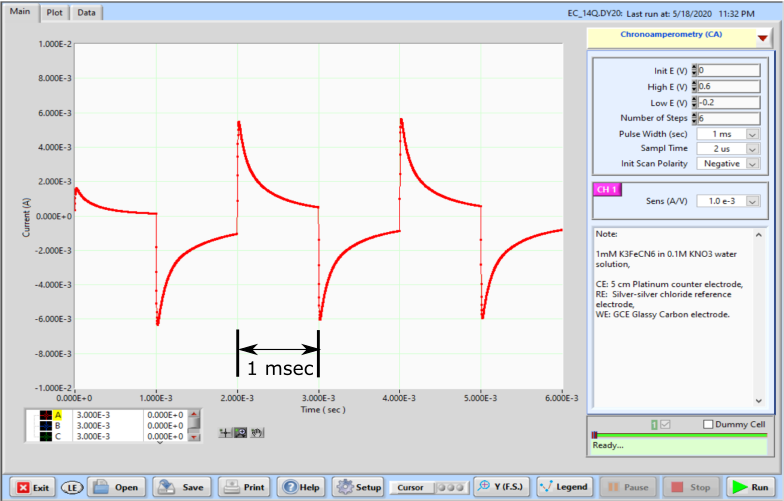•